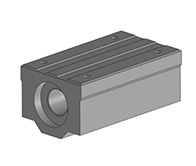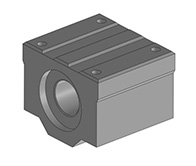Metal Parts Products Manufacturers at China

# Machining the ball screw linear bearing box unit

Product Item: Machining the ball screw linear bearing box unit
Category: Turning Parts
Main parameters of ball screw thread
1) outer diameter d (large diameter) (D) - the diameter of the imaginary cylindrical surface that coincides with the external thread crest - also known as the nominal diameter
2) Inner diameter (small diameter) d1 (D1) - the diameter of the imaginary cylindrical surface that coincides with the root of the external thread, and the calculated diameter of the dangerous section in the strength calculation
3) Medium diameter d2 - the diameter of the imaginary cylindrical surface where the tooth thickness is equal to the interdental width in the axial section, approximately equal to the average diameter of the thread d2 ≈ 0.5 (d + d1)
4) Pitch P ——The axial distance between two adjacent points on the busbar of the medium-diameter cylindrical surface
5) Lead (S) - the axial distance between two points of the adjacent two teeth on the same spiral line on the busbar of the medium-diameter cylindrical surface
6) Number of lines n ——The number of thread spirals is generally convenient for manufacturing n≤4 pitch, lead, and number of lines: S=nP
7) Spiral angle ψ - the angle between the tangent of the helix on the cylindrical surface and the plane perpendicular to the axis of the helix.

• PRODUCT DETAIL
8) tooth angle α - The angle between the two sides of the thread profile in the axial plane of the thread
9) Tooth angle β—the angle between the side of the thread profile and the vertical plane of the thread axis. The main geometric dimensions of various threads of symmetrical teeth (except for rectangular threads) can be found in the relevant standards. - The nominal size is the outer diameter of the thread and the pipe thread is approximately equal to the inner diameter of the pipe.
The self-locking condition of the spiral pair is: The thread lift angle is less than or equal to the equivalent friction angle of the screw pair.
The transmission efficiency of the screw pair is: The ratio of effective work to input work when the nut is rotated one revolution.
Overcoming the circumferential force required for the uniform increase of the axial force Q

 SCS-LUU- For the outer wheel separation type and the inner wheel rotation.      - For use where it is necessary to rotate the inner wheel. SCS-UU﻿
﻿